﻿ Research on Prosumer Combining Household Electricity, Electric Vehicle Home Charging and Photovoltaic Power GenerationPublications are Open
Access in this journal
Article Versions
Export Article
• Normal Style
• MLA Style
• APA Style
• Chicago Style
Research Article
Open Access Peer-reviewed

### Research on Prosumer Combining Household Electricity, Electric Vehicle Home Charging and Photovoltaic Power Generation

Jiashu Wang , Ying Wang, Zetian Zhong, Gang Ma
American Journal of Electrical and Electronic Engineering. 2019, 7(1), 18-23. DOI: 10.12691/ajeee-7-1-4
Received April 11, 2019; Revised May 19, 2019; Accepted June 13, 2019

### Abstract

Nowadays, the situation of environmental pollution is increasing, energy is getting more and more tight, and traditional fossil energy can no longer meet people's needs. Solar energy has received extensive attention because of its clean and safe characteristics. This paper combines the three-point practical model of photovoltaic(PV) power generation, the Bernoulli model of electric vehicle(EV) home charging and the Weibull distribution model of household electricity to form the Prosumer probability distribution model, and analyze the daily power fluctuations in different scenarios. Then based on the IEEE33 node system, considering the access of the PV system, the influence of the Prosumer access on the voltage offset and network loss of the distribution network node at different times is analyzed. The results show that the family access to the PV system can effectively reduce the voltage offset and the total network loss of the line.

### 1. Introduction

In 2018, China's new PV installed capacity exceeded 43GW, and the cumulative installed capacity exceeded 170GW. PV power generation showed a rapid development trend, and the installed capacity of household PV reached 8GW 1. In order to scientifically analyze the output characteristics of rooftop PVs and achieve reliable application of PVs, relevant scholars have done a lot of research. In 2 for the voltage overrun and voltage fluctuation of the household PV access distribution network, the reactive power control strategy of Mamdani fuzzy inference effectively suppresses the voltage fluctuation. In the aspect of demand side management, reference 3 proposes an autonomous energy consumption scheduling algorithm, which reduces the peak load by scheduling the operation of the load, and solves the reverse power flow problem in which the power generation of the PV device is greater than the total load of the household. In 4 a nonparametric model is proposed to solve the variation law of PV power generation by using the solution set and conditional kernel density estimation technique, and the feasibility of the model is verified. In 5 the household PV system and energy storage system are connected to the power grid, and an optimized storage control algorithm is proposed. By solving the convex optimization problem with polynomial time complexity, the optimal control of charge and discharge of the control storage system is realized. Studies have shown that the PV output model conforms to several distributions such as normal distribution, beta distribution, and Weibull distribution 6, 7.

At the same time, EVs are gradually replacing traditional fuel vehicles because of their clean and environmentally friendly features. Therefore, it is of practical significance to predict the load of EVs. In 8 the EV charging station and the distribution network are jointly planned, and a mixed integer nonlinear programming model is established. The segmentation linearization is used to deal with the network loss, which improves the economic and reliability of the planning. In 9 aiming at improving load stability and optimizing configuration economy, based on the disordered charging model of EVs, the distributed power supply installation cost, operation and maintenance cost, EV charging cost, and micro-grid total load fluctuation are established. Multi-objective planning model. Reference 10 is aimed at the orderly charging of EVs in residential areas, combining online control with offline optimization, and the charging load is less than the maximum allowable power through the ordered charging controller. In 11, considering the two factors of the end time and distance of the trip, the space-time distribution model of the EV was established. The Monte Carlo method was used to simulate the charging load of EVs in different seasons and penetration rates. Reference 12 predicts the load of EVs based on the idea of travel chain, considers the charging requirements of the traveler and the location of the charging station, and establishes the charging station site selection model through the immune algorithm, which improves the rationality of the charging station site selection.

China's household PV industry has developed rapidly. At the end of 2017, household PV reached 500,000 sets 13. EVs are also becoming more and more popular, and their charging behavior has a significant impact on the operation of the distribution network. Therefore, it is of practical significance to combine small household PV power generation with EV electricity. With the development of the power grid, traditional power consumers not only consume electrical energy, but also produce and store electrical energy, such users are defined as Prosumer 14. Reference 15 proposed a charge and discharge scheduling method for EVs for home users of Prosumer. The central scheduling program establishes a hybrid optimization model to improve the interests of consumers. Reference 16 studied the interaction between household electricity and EV charging, and the heat generated by the cogeneration unit to charge the EV to meet the heat demand of the house. Reference 17 takes the city of Westminster in London as an example to study household electricity, EV home charging and distributed PV power generation. Research shows that the PV power generation is all used for household load, and the total power generation will not exceed the consumption. In summary, there are few studies on household Prosumer in China, and most of them are research on the charging and discharging of PV power generation systems or EVs. This paper first establishes three independent models of household electricity, PV power generation, and EV home charging, and then combines the three probability models to form a single power unit of the Prosumer. Then the power flow characteristics of the Prosumer are analyzed, and the influence of the access of the PV system on the load fluctuation is compared. Finally, the 33 voltage node model is used to analyze the influence of Prosumer access on distribution network voltage and network loss. The results show that after the introduction of the power unit of the Prosumer, the electricity generated by the household PV system can meet some household electricity during the day, and can effectively reduce the voltage offset and the total network loss of the line.

### 2. Prosumer Model

With the expansion of the scale of PV systems, users are no longer simply power consumers, but gradually become Prosumers. This paper combines household PV power generation, EV home charging and household electricity to form the Prosumer family unit, combining the probability distribution model of power production and consumption, quantifying the problem of power consumption and production mismatch, reducing user costs and reducing the daily load fluctuates.

The model framework is shown in Figure 1. The central framework is a stochastic variable of the user's electricity/generation generated by the random variable X. The model is the sum of the random variables of the household electric XH, the EV home charging XEV, and the PV power XPV. Here, electricity is positive and power generation is negative. If the value of X is positive, it indicates that the user's power consumption is greater than the power generated by the PV. At this time, it needs to be powered by the large power grid. If the value of X is negative, it indicates that the electricity generated by the PV is redundant with the power used by the user. Excess electrical access to the distribution network.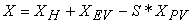(1)

Where S is the area of the household PV panel, because XPV is defined as the PV cell power generation (W/m2) with a module area of 1 m2.

• Figure 1. Prosumer model
2.1. Household Electricity Model

Household electricity is random, and electricity usage is not the same at different times and seasons. At present, Weibull distribution and lognormal distribution are generally used to simulate household electricity use 18. This paper uses the Weibull probability density function to model household electricity. The model in this article is based on two assumptions:

(1) At any time, the household's electricity demand is a random result.

(2) The probability of all possible power usage can be approximated by a continuous function.

Weibull function uses the maximum likelihood method to estimate hourly, daily, weekly, and yearly data categories.

The result has a total of 24*7*12=2016 distributions. The probability density function X of the Weibull random variable is defined as: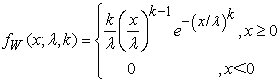(2)

Among them, k＞0 is called a shape parameter and λ＞0 is called a scale parameter. The integral form of the Weibull random variable W is: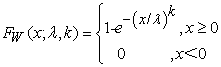(3)

When k=1, the Weibull distribution obeys the index distribution; when k=2, it obeys the Rayleigh distribution. The mean (μ) of the distribution is: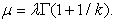(4)

The variance of the distribution (σ²) is: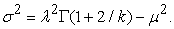(5)

Here Γ(x) is the Γ function.

In this paper, the EV charging load model is based on the time-space characteristic analysis method of the travel chain, and the Monte Carlo method is used to predict the charging load of EVs in one day 19. User activity types are divided into five categories: home (H), work (W), shopping (SE), social leisure, and other things (O) 20. The resulting travel chain is shown in Figure 2. Figure 2 assumes that the user's daily itinerary is the above five categories, the EV is charged at these five destinations, and the end time of each trip is random. The fitting is performed by the Weibull function, and the probability density is as follows: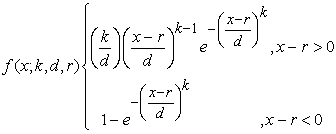(6)

Where x is a random variable, k is a shape parameter, d is a scale parameter, and r is a position parameter. d is related to the average of the end of the trip, min. d approximates a lognormal distribution with a probability density of: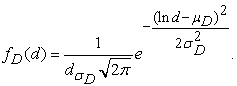(7)

Among them, μD represents the expected value of the stroke; σD represents the standard deviation of the stroke.

• Figure 2. The structure of home-based travel chain
2.3. PV Power Generation Model

PV power generation uses a three-point practical model 21, which first predicts solar radiation, the predicted radiation intensity is then derived from the photoelectric conversion rate to obtain the PV output model. Let the parabola y=ax2+bx+c, the two points of the sun rise and sunset are (x1, 0), (x2, 0). Set the peak of sunshine intensity at 12 o'clock, corresponding to the parabola vertex (xmax, ymax). From these three points, the PV power generation model can be obtained.

The two points of rising and sunset can be obtained by the solar height angle calculation formula: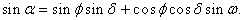(8)

Among them, α is the solar altitude angle; φ is the local geographic latitude, the southern hemisphere is negative, the northern hemisphere is positive; δ is the declination angle; ω is the solar time angle at that time. The solution of the peak is obtained by the ASHRAE model, and its expression is: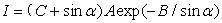(9)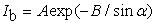(10)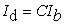(11)

Where A is the apparent solar radiation at atmospheric mass; B is the extinction coefficient of the atmosphere; C is the scattering radiation coefficient; I is the total solar radiation value of the horizontal plane; Ib is the horizontal direct radiation value; Id is the horizontal plane scattering radiation value. A takes 1107W·m-2 , B takes 0.201, and C takes 0.122.

### 3. Prosumer Daily Power Fluctuation

3.1. EV Charging in Prosumer

The Monte Carlo simulation described in Section 2.2 predicts the load curve of the average charging power of an EV over time, as shown in Figure 3. As can be seen from the figure, the peak charging period is from 4 pm to 10 pm, the peak value is about 6 pm, and the size is about 4500W. At this time, the user ends the day's journey and returns home to charge the EV.

• Figure 3. EV charging daily load forecast curve
3.2. PV Power Generation in Prosumer

The solar radiation is selected from the intensity of sunshine in Nanjing, China (32°north latitude) on August. PV power generation is based on a solar radiation intensity conversion efficiency of 14% and an area of 50 m2. The curve of solar irradiance over time is obtained by the method of Section 2.3, as shown in Figure 4. It can be seen from the figure that the sun rises around five o'clock, falls around 6 o'clock in the afternoon, and reaches a maximum at 12 o'clock. The output of PV depends on the intensity of sunlight, the conversion efficiency of sunlight intensity and the area of PV panels, as shown in the following formula: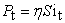(12)

Where Pt is the output of the PV power station at time t, W;η is the energy conversion efficiency; S is the roof PV panel area, m2; it is the sunshine radiation value at time t, W/m2. The PV output simulation results are shown by the dotted line in Figure 5.

• Figure 4. Solar irradiance curve
3.3. Daily Power Forecast for Each Part of the Prosumer

Figure 5 is a graph showing the predicted power of household electric power, PV power, and EV charging load, with a time interval of one hour. It can be seen from the figure that the household electrical power has a large randomness and a large fluctuation, but the peak period of power consumption is concentrated between 7pm and 9pm. PV power generation is concentrated from 8 am to 4 pm, most of which generate less power than EV, but more than household electricity. This shows that most of the daytime household electricity can be provided by the electricity generated by the PV panels; from the afternoon to the evening, it needs to be powered by the grid. If the PV power generation system is added to the energy storage device, the excess energy of the daytime PV power generation can be stored for household electricity and EV charging after sunset.

• Figure 5. Daily load forecasting chart for each part of Prosumer

Figure 6 shows the daily fluctuation curve of the electricity/power generation in different usage scenarios, including electricity/power generation with or without EVs and PV access. The thin solid line indicates the situation when the household accesses the PV system. The negative value during the day is because the amount of PV power generated during the day is more than the amount of electricity used by the user, reaching the valley at noon, and the sunshine intensity is the strongest. The morning fluctuations are minimal, because there is no electricity generated by PVs during this time, and household electricity is also less. The dotted line is the fluctuation curve of the total power consumption of the household after the EV is connected. Since there is no access to the PV system, the ripple curve is all above zero. EVs are mainly concentrated at night charging, which increases the amount of electricity used at night. The peak power consumption is around 4500W. The star drawing line is a power fluctuation curve combined with EV charging and household electricity after joining the PV system, and its fluctuation curve is in the middle of the two curves. Compared with not accessing an EV, it fluctuates less during the day and fluctuates greatly during the night. Compared with not accessing the PV system, it fluctuates greatly during the day and has a higher coincidence between the night and the early morning of the next day. Because the PV system does not contribute after the sunset, and the power used by the user is provided by the grid.

• Figure 6. Daily fluctuation curves in different scenarios

• Figure 7. IEEE33 distribution network system topology

In this paper, the influence of Prosumer access on node voltage and network loss of distribution network is analyzed by means of IEEE33 node distribution system. The topology is shown in Figure 7, and node 1 is the balance node.

4.1. Impact of Prosumer Access on Node Voltage

When the power unit of the Prosumer is connected to the distribution network, the voltage offset will occur. The voltage offset will have a bad influence on the power equipment, and even cause a large-scale power outage, which will affect the residents' lives and safety. Therefore, this paper analyzes the influence of Prosumer access on the voltage deviation of the distribution network from both time and space through Matlab/Simulink simulation. Figure 8 shows that when the PV is not connected, the voltage offset fluctuates between 0 and 10%. When the time is fixed, the voltage offsets of different nodes are different. Taking 10 o'clock in the morning as an example, the 18-node voltage offset is the largest, reaching 7.18%. When the node is fixed, the voltage offset changes with time. Taking 10-node as an example, the voltage offset at 24 o'clock is the largest, which is 1.78%. Figure 9 shows the situation when accessing PV. It is obvious that the voltage offset is much reduced, fluctuating between -1% and 5%. At 10 o'clock in the morning, the 18-node voltage offset is the largest, at 4.03%. The 10-node have the largest voltage offset at 24 hours, which is 1.12%. It can be seen that the access to the PV system in the home can effectively reduce the voltage offset.

• Figure 8. Time-space characteristic curve of voltage offset of Household+EV access distribution network
• Figure 9. Time-space characteristic curve of voltage offset of Household+EV-PV access distribution network
4.2. Impact of Prosumer Access on Network Loss

The power loss of the power system is an important technical and economic indicator, which has practical significance for the calculation of the total network loss of the line. The impact of Prosumer access on distribution network loss is considered from two aspects of time and space. Figure 10 shows the relationship between the total line loss and the time and voltage nodes when the PV system is not connected. It can be seen from the figure that the total network loss of the line reaches 0.45 MW. When the time is fixed, the network loss rate increases with the increase of the node number. When the node is fixed, the network loss of the line fluctuates up and down, which is related to the residents' electricity usage behavior. Figure 11 shows the total network loss of the line after access to the PV system. It can be seen that when the home is connected to the PV system, the network loss of the line is significantly reduced. Taking 10 o'clock in the morning as an example, 15-node were selected, and the network loss was reduced from 0.2713 MW to 0.2356 MW. If the network loss is too large, it will have a negative impact on the distribution network. Therefore, reducing the network loss by accessing the PV system is beneficial to the operation of the distribution network.

• Figure 10. Time-space characteristic curve of Household+EV access distribution network loss
• Figure 11. Time-space characteristic curve of Household+EV-PV access distribution network loss

### 5. Result and Discussion

This paper combines household electricity, EV home charging and PV power generation to form the Prosumer model, which improves user economy and reduces household load fluctuations. By simulating the actual load fluctuations of the electricity/power generation when the household electricity is combined with the EV charging and PV power generation, it can be seen that the electricity generated by the household PV system during the day can meet the household electricity demand most of the time and at noon, the amount of PV power generation exceeds the household electricity consumption. Therefore, it is considered to access the energy storage system to store the remaining electrical energy of the daytime PV system for nighttime use. And based on the IEEE33 node distribution network model, the influence of Prosumer access on distribution network voltage offset and network loss is analyzed from both space and time. The results show that the family access to PV system can effectively reduce the voltage offset and the total network loss of the line. Therefore, in practice, it is beneficial for residents to add PV system to the roof for themselves and the grid. Since household electricity and PV power generation have strong randomness, the prediction of this model has certain errors, and the model can be improved by refining the dependencies of various parts.

### Acknowledgements

This research was supported by Postgraduate Research & Practice Innovation Program of Jiangsu Province (KYCX19_0809).

### References

  Wang Bohua, "Development of Photovoltaic Industry in China: Review in 2018 and Prospect in 2019," Available: http://www.nengyuanjie.net/article/22816.html, [Accessed: Jan. 17, 2019]. In article  Xu Guyang, Tang Wei, Cai Yongxiang, Sheng Wanxing, Yu Lingfeng, "High-Ratio Household PV Reactive Power Control Strategy for Low Voltage Distribution Network Based on Fuzzy Logic," Automation of Electric Power Systems, 41(23): 89-95, 2017. In article  Xia K , He Z , Yuan Y," An arc fault detection system for the household PV inverter according to the DC bus currents," in International Conference on Electrical Machines & Systems, IEEE, 2016. In article View Article  Jin Liming, Zhou Ning, Feng Li, Fan Fei, Zhao Yuan," Study on time series probability model of PV power generation system," Electric Power Construction, 37(07): 27-32, 2016. In article  Wang Y, Lin X, Pedram M," A Near-Optimal Model-Based Control Algorithm for Households Equipped With Residential PV Power Generation and Energy Storage Systems," IEEE Transactions on Sustainable Energy, 7(1): 77-86, 2016. In article View Article  Zhang Wei, Kang Chongqing, Zhang Ning, Huang Yuehui, Liu Chun, Xu Jianfei, “Medium and Long Term Random Characteristic Analysis of Solar Photovoltaic Power Generation,” Automation of Electric Power Systems, 38(6): 6-13, 2014. In article  Wu Zhenwei, Jiang Xiaoping, Ma Huimeng, Ma Suliang, Study on Fluctuation Characteristics of PV Output on Multiple Time Scales,” Modern Electric Power, 31(1): 58-61, 2014. In article  Jia Long, Hu Zechun, Song Yonghua, Ding Huajie, "Study on joint planning of energy storage and EV charging station and distribution network," Journal of Electrical Engineering of China, 37(01): 73-84, 2017. In article  Ding Ming, Shi Shengliang, Pan Hao, Wang Min, Yao Yuliang," The AC/DC Hybrid Microgrid Planning with EV Charging Load," Automation of Electric Power Systems, 42(01):32-38+81,2018. In article  Qi Xianjun, Li Dongwei, Ji Shuyan, "Power-limited orderly charging control strategy for residential EVs," Power grid technology, 40 (12): 3715-3721, 2016. In article  Chen Lidan, Nie Yongquan, Zhong Qing," EV Charging Load Forecasting Model Based on Travel Chain," Journal of Electrical Technology, 2015, 30 (04): 216-225. In article  Xu Qingshan, Cai Tingting, Liu Yujun, Yao Liangzhong, Zeng Pingliang," Location planning of EV charging station considering driver behavior and travel chain," Power system automation,40 (04): 59-65+77, 2016. In article  Chen Dongpo,"Review and Prospect of China's PV Market in 2017-2018," Electronic Products World, 25(04):11-13, 2018. In article  Chukwuka V, Nazari M H, Grijalva S, "Enhancing communications reliability in prosumer-based smart grid using MIMO techniques," Power Systems Conference, 2016. In article View Article  Abbas M, Kiseon K, "Low-Complexity Charging/Discharging Scheduling for EVs at Home and Common Lots for Smart Households Prosumers," IEEE Transactions on Consumer Electronics, 2018. In article  Vialetto G, Noro M, Rokni M, "Combined micro-cogeneration and EV system for household application: An energy and economic analysis in a Northern European climate," International Journal of Hydrogen Energy, 2017. In article View Article  Munkhammar J, Bishop J D K, Sarralde J J, "Household electricity use, EV home-charging and distributed PV power production in the city of Westminster," Energy & Buildings, 86: 439-448, 2015. In article View Article  Munkhammar J, Rydén J, Widén J," Characterizing probability density distributions for household electricity load profiles from high-resolution electricity use data," Applied Energy, 135: 382-390, 2014. In article View Article  He Ruotai, Liu Cheng,Wang Ying, Zhao Rui., "Analysis of time and space characteristics of the influence of disordered charging of EVs on distribution network based on travel chain," Electrical Application, 37(21):29-35, 2018. In article  Chen Jingpeng, Ai Wei, Xiao Fei, "Planning of EV Charging Station Based on User Travel Demand," Electric Power Automation Equipment, 36(6): 34-39, 2016. In article  XU Qingshan, ZHAI Haixiang, YAN Haihong, "Establishment and Feasibility Study of Practical Model of Solar Radiation," Journal of Solar Energy, 32(8): 1180-1185, 2011. In article

Published with license by Science and Education Publishing, Copyright © 2019 Jiashu Wang, Ying Wang, Zetian Zhong and Gang MaThis work is licensed under a Creative Commons Attribution 4.0 International License. To view a copy of this license, visit http://creativecommons.org/licenses/by/4.0/